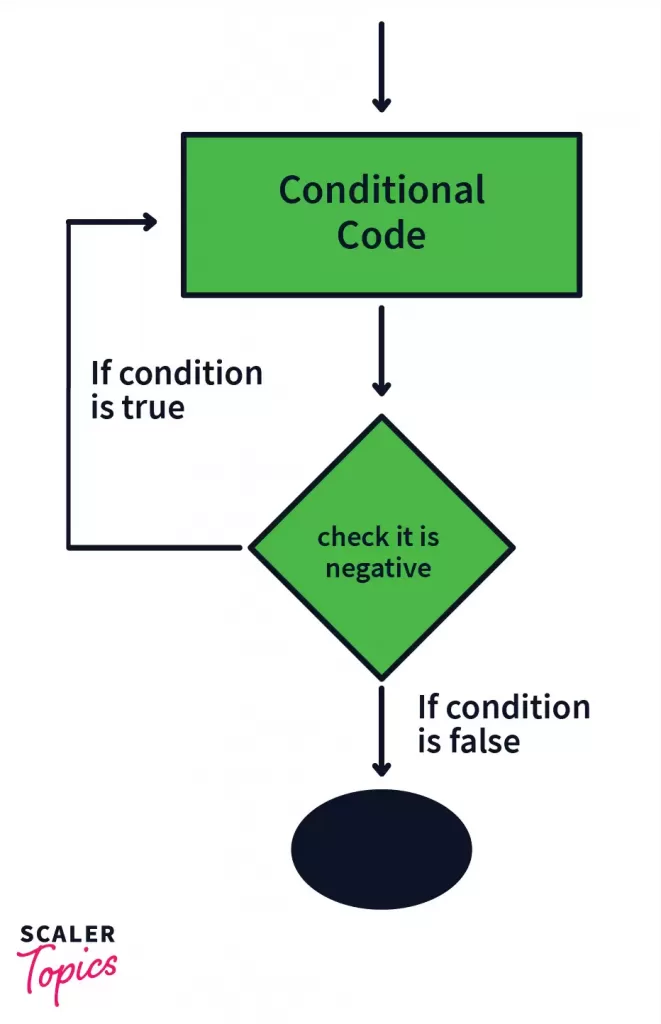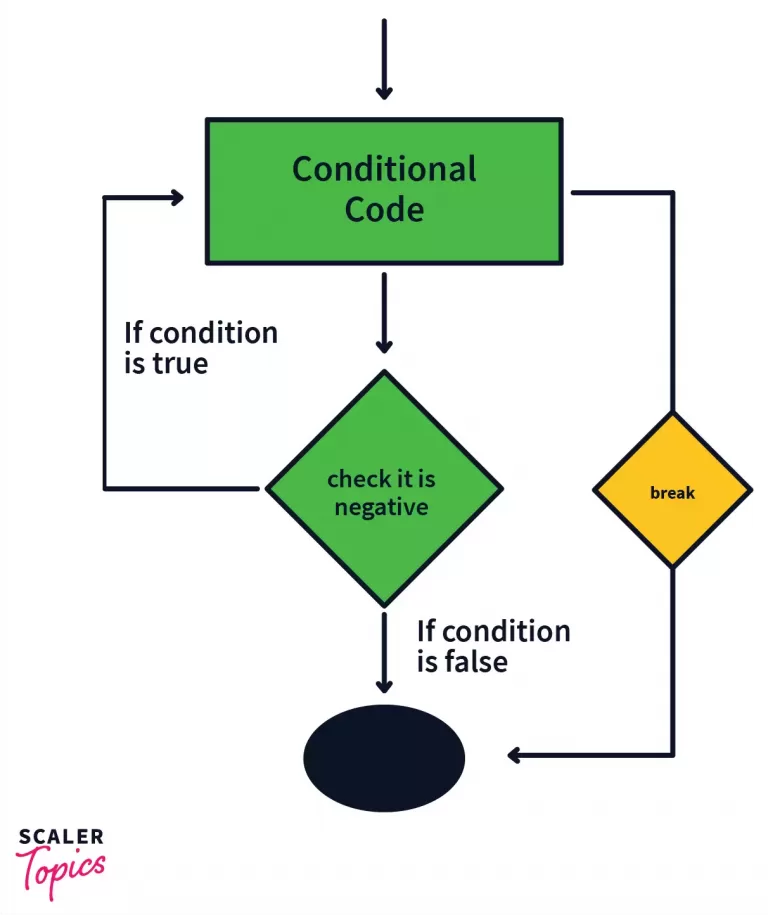# Break Statement in Java

Challenge Inside! : Find out where you stand! Try quiz, solve problems & win rewards!
Video Tutorial## Overview

Break statements in java are used to end the ongoing process. They can be used to terminate for loops, while loops and do-while loops. They are also used to come out of the switch block once the matching case has been found.

## Introduction to Break Statement in Java

The break statement in the programming world is used to terminate or end an ongoing process. Let us understand this deeply with the help of a real-life example.

Say we want to fill a bottle with water, so we turn on the tap, and the bottle starts getting filled with water. As soon as the bottle gets completely filled we turn the tap off.

Now if we observe closely, we see that the bottle getting filled was the ongoing process that we terminated as soon as the bottle got filled, that is once our work was done.

This is exactly what the break statement does, now let us dive into the working of the break statement in java.

## Flowchart of Break Statement in Java

We’ve already seen above that a break statement helps us to end or terminate an ongoing process, by breaking the current flow of the program, but let us now understand how that happens.In the picture given above, we see that the flow of control takes places like this:

1. Control goes to Conditional Code.
2. Control then moves to the Condition.
3. The condition is checked to see whether it is True or False.
4. If the condition is True, control jumps back to step 1.
5. If the condition is False, control goes to the black oval denoting the end of the program

Now if we add a break statement in the Conditional code section, the working changes completely. Let us see how.1. Control goes to Conditional Code.
2. The control encounters the break statement which forces it to move out of the Conditional code structure and takes it to the black oval denoting the end of the program.

In this example, we can see that because of the break statement, the control was taken directly to the end of the program, instead of being taken to the Condition section.

This means that the break statement terminated the execution of the Conditional Code and took the control out of the code structure, straight away to the black oval denoting the end of code.

The break statement in java is given by the break keyword, the syntax is given as:

Now let us see how and where we can use the break statements in java.

## Use Cases of Break Statement in Java

Break statement in java is used in several cases. Like for-loop, while-loop, do-while loop, and with switch-case statements. Let us see and understand each one of them.

### 1. For Loops

• #### Single For Loop

Output:

Explanation:

In the above example, we see that the for loop starts from 1 and keeps iterating till it reaches 9. Inside the loop, we have a print statement that prints i and we have an if-condition that contains the break statement. This break statement will execute only when i becomes equal to 4.

Let us see the dry run of the given code.

• #### Nested For Loops

Output:

Explanation:

In the above example, we see that there are two for loops, one inside the other. Let us name the i loop as outer and the j loop as inner.

The outer loop starts iterating from 1 till it reaches 3. For every iteration of the outer loop, the inner loop iterates from 1 to 6.

Inside the inner loop, we have a print statement for j and we also have an if-condition that contains a break statement. This condition holds only when i, becomes equal to 4.

Let us see the dry run of the given code. We will try to understand how a single iteration of the outer loop works.

The same process repeats for i=2 and 3, after which the outer loop ends and the statement “End of outer loop” gets printed.

If we observe the above code we see that each time the break statement terminates only the inner loop whereas the outer loop was unaffected throughout the execution of the program.

An important point to note here is that the break statement always breaks or terminates the execution of the innermost for loop that it is present in.

In the picture given above, once the break statement is encountered in the innermost loop, it breaks from that loop and goes to the line just outside it.

• #### Using Lables

Till now we have seen how to break from nested for loops. But the problem was that we could break only from the innermost loop.

What if we wanted to break from the outermost loop or a loop of our choice? For this, we use the label keyword.

Syntax: break label_name;

Output:

Explanation:

In the above example, we see that there are two for loops, one inside the other. Let us name the i loop as outer and the j one as inner.

The outer loop starts iterating from 1 till it reaches 3, and for every iteration of the outer, the inner loop iterates from 1 to 6.

Inside the inner loop, we have a print statement for j and we also have an if-condition having a break statement, that holds only when i, becomes equal to 4.

Since here, the ith loop is labeled as outer, the control moves out of the outer loop as well and it goes to the print statement “End of outer loop”.

An important point to note here is that if the break statement is used with a label, then the control moves out of the code structure having that label. Let us understand the cases with the following picture.

In the above picture we can see that when the first condition present inside the innermost loop becomes true, the break inner statement is encountered. This takes the control out of the code structure that is labeled as inner, which in this case is the middle loop.

In case the second condition becomes true, the break statement takes the control out of the code structure labeled as outer, which is the outermost or parent loop.

This is how the break statement works with labels in java.

### 2. While Loops

The working of the break statement in the while loop is the same as in the case of for loops, which we’ve already seen above.

• #### Simple While Loop

Output:

Explanation:

The above example works exactly like a normal for loop. The loop starts iterating from 1 to 9. When the value of i becomes 4, the if-condition becomes true.

The break statement then terminates the loop and moves to control to the print statement “End of loop”.

• #### Nested While Loop

Here also the working is the same as in the case of nested for loops and the same rule applies that in the case of nested while loops the break statement terminates the innermost loop that it is present in, if it is used without a label.

In case we use labels, the working remains the same as in the case of for loops. Let us now see the working of nested while loops with labels.

Output:

Explanation:

The outer loop starts iterating from 1 till it reaches 3, and for every i, the inner loop iterates from 1 to 6.

Inside the inner loop, we have a print statement for j and we also have an if-condition having a break statement, that holds only when i, becomes equal to 4.

It looks for the ‘outer’ label in the program and takes the control out of the code structure labeled as ‘outer’. It moves directly to the print statement: “End of loop”.

### 3. Do While

We’ve already seen the working of break statements in two loops, i.e., for and while loop. In the do-while loop also the break statement functions in the same manner. Let us see a different example in this case.

Output:

Explanation:

In the above example, we see that the for loop starts iterating from 1 and keeps on iterating because of the given condition → (i>0) . This condition can never become false.

So this loop is called an Infinite loop since it goes on executing till infinity and never stops. In such cases, the break statement helps us in breaking or terminating the loop at some point.

Here we see that when i becomes 6, the if-condition becomes true and then the break statement terminates the loop, taking the control directly to the print statement “End of loop”.

### 4. Switch Case

Now that we’ve seen how break statements work with loops, it’s time to see how they work with switch-case statements.

Let us understand it with the help of an example.

Output:

Explanation:

In the above example, the switch statement takes in a parameter whose value is 4. Then it tries to match it with the different cases.

When case 4 matches with the parameter value, “Four” gets printed, and then the break statement is encountered. This break takes the control out of the switch case construct directly to the print statement of “Out of switch case”.

Had the break statement not been there, the other case blocks after case 4 would also get executed. Let us see this with another example.

Output:

Explanation:

In this example, once the parameter of switch matches with case 3, “Three” gets printed. But in this case, there is no break statement in the case block, because of which the subsequent case blocks also get executed, which means “Four” and “Invalid” also get printed.

Thus an important point to note here is that in the absence of break statements, once the parameter matches with a case value, that case block and all the other case blocks below it get executed until a break statement is encountered.

## Conclusion

• Break statements help us in terminating a loop or breaking from a switch-case construct.
• In the case of nested loops, break statements terminate the innermost loop they are present in.
• The working of break statements for all types of loops is the same.
• In the case of nested loops if we wish to break from a specific loop, then we use labels. We can then name each loop and can terminate whichever loop we want to.
• In switch case construct, the break statements help in moving out of the code structure once the case block has been executed.
• In the absence of break statements, once a case block has been executed, all the other cases below it also get executed until a break statement is encountered.